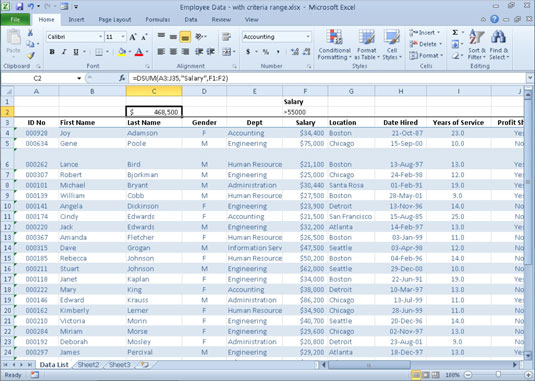##### Excel 2010 All-in-One For DummiesYou can use Excel 2010's database functions to calculate statistics, such as the total, average, maximum, minimum, and count in a particular database field when the criteria that you specify are met. For example, you could use the DSUM function in an Employee database to compute the sum of all the salaries for employees who were hired after January 1, 2000, or you could use the DCOUNT function to compute the number of records in the database for the Human Resources department.

The database functions all take the same three arguments as illustrated by the DAVERAGE function:

`=DAVERAGE(database,field,criteria)`

The arguments for the database functions require the following information:

• Database specifies the range containing the database. It must include the field names in the top row.

• Field is the argument that specifies the field whose values are to be calculated by the database function (averaged in the case of the DAVERAGE function). You can specify this argument by enclosing the name of the field in double quotes (as in "Salary" or "Date Hired"), or you can do this by entering the number of the column in the database (counting from left to right with the first field counted as 1).

• Criteria is the argument that specifies the address of the range that contains the criteria that you're using to determine which values are calculated. This range must include at least one field name that indicates the field whose values are to be evaluated and one cell with the values or expression to be used in the evaluation.

The following table lists the database functions available in Excel along with an explanation of what each one calculates.

The Database Functions in Excel 2010
Database Function What It Calculates
DAVERAGE Averages all the values in a field of the database that match the criteria you specify.
DCOUNT Counts the number of cells with numeric entries in a field of the database that match the criteria you specify.
DCOUNTA Counts the number of nonblank cells in a field of the database that match the criteria you specify.
DGET Extracts a single value from a record in the database that matches the criteria you specify. If no record matches, the function returns the #VALUE! error value. If multiple records match, the function returns the #NUM! error value.
DMAX Returns the highest value in a field of the database that matches the criteria you specify.
DMIN Returns the lowest value in a field of the database that matches the criteria you specify.
DPRODUCT Multiplies all the values in a field of the database that match the criteria you specify.
DSTDEV Estimates the standard deviation based on the sample of values in a field of the database that match the criteria you specify.
DSTDEVP Calculates the standard deviation based on the population of values in a field of the database that match the criteria you specify.
DSUM Sums all the values in a field of the database that match the criteria you specify.
DVAR Estimates the variance based on the sample of values in a field of the database that match the criteria you specify.
DVARP Calculates the variance based on the population of values in a field of the database that match the criteria you specify.

The Database functions are too rarely used to rate their own command button on the Ribbon's Formulas tab. As a result, to use them in a worksheet, you must click the Function Wizard (fx) button on the Formula bar, click Database in the Select a Category drop-down list box, and then click the function to use — or you can type the Database function directly into the cell.

The example shown in the figure below illustrates the use of the database function DSUM. Cell C2 in the worksheet shown in this figure contains the following formula:Using DSUM to total the salaries over \$55,000 in an Employee database.
`=DSUM(A3:J35,"Salary",F1:F2)`

This DSUM function computes the total of all the salaries in the database that are above \$55,000. This total is \$468,500, as shown in cell C2, which contains the formula.

The database argument is the range A3:J35, which contains the entire database, including the top row of field names. The field argument is "Salary" because this is the name of the field that contains the values to total. Finally, the range F1:F2 is the criteria argument because these two cells contain the criteria range that designate that only the values exceeding \$55,000 in the Salary field are to be summed.